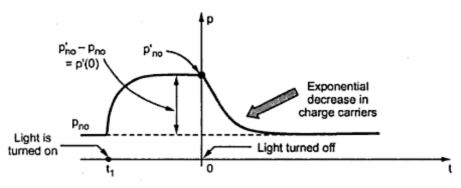### Generation and Recombination of Charges

In a pure semiconductor, the number of holes (p) is equal to the number of electrons (n). Due to increase in temperature or due to light incident on the semiconductor, the number of electron-hole pair increases. This is called generation of charges.
While few other electron-hole pairs disappear which is due to recombination of charges. The recombination is a process of falling of a free electron into an empty covalent bond, due to which the corresponding electron-hole pair gets disappeared. In a recombination an electron moves from a conduction band to a valence band.
The carrier lifetime is defined as the mean time for which, a charge carrier exists before recombination. After recombination the charge carriers vanish. For an electron it is denoted as τn and for a hole it is denoted as τp.
Consider n type semiconductor bar having equilibrium concentrations of electrons and holes as nno and pno.
At t = t1 , the bar is illuminated by the light of proper frequency, then the new electron-hole pairs are generated, uniformly throughout the bar.
The new concentrations of electrons and holes are n'no and p'no, when equilibrium situation is reached. Then the excess charge carriers of holes and electrons are equal and given by,

This is shown in the Fig. 1.Fig. 1 Variation of hole concentration against time
Though the number of electrons and holes generated are same in number, in n-type bar the electrons are large in number. Thus excess electrons hardly affects the behaviour of the bar. But the excess holes have significant effect on the number of holes present, which are minority in number.
Note : Thus the radiation affects the number of minority charge carriers singificantly, which needs to be analyzed. It hardly affects the majority charge carrier concentration.
Now at t = 0, the light is switched off where the equilibrium is reached. Then the concentration of charge carriers returns so the original value exponentially. Thus the excess carrier concentration decreases to zero exponentially, as shown in the Fig. 1.
1.1 Mathematical Analysis
The rate of change in hole concentration is the rate of generation of holes minus the rate of recombination.
The rate of generation totally depends on the temperature and is constant for constant temperature.
While the rate of recombination is decided by the mean life time of the holes τp.
...      Rate of recombination of holes = pn
Under steady state condition, dpn/dt = 0 hence the hole concentration again becomes pno.
Rate of generation of holes = pno
But the excess carrier concentration is pn - pno and is a function of time denoted as p'(t).
This is the required differential equation governing the behaviour of excess charge concentration.
The solution of the equating (5) gives,
The equation (6) shows that as t ∞, p'(t) 0 and thus the excess charge concentration reduces to zero exponentially, with a time constant equal to τp.
The important remarks are,
1. Measurement of τ is is important as it directly influence the characteristic of diodes and transistors.
2. Usually the life time τ ranges between 1 to 1000 μs, but it depends on impurity concentration and temperature of material.
3. Practically for manufacturing special devices, the recombination agent is used to control the rate of recombination. The gold is popularly used as a recombination agent.